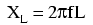#12 years ago

REMEMBER, here frequncy is generally 50 Hz so calculate the effectivwe resistance (reactance) of the inductor by the given formula ..

XL=2x3.14x50x5/100;      XL=15.71Ω

then the parallel combination and finally the seriers conbination ...

1/R=1/10+1/15.71;      R=0.163653723...;                 then  R'=10+10+0.163653723 R'=20.163653723

the totyal resistane comes,divide it by 6 volts and the answer is 0.3 A approx  I=V/R     I=6/20.163653723        I=0.297565118.....  approx 0.3 A   Ans.

If you like it then please reply ....